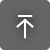TL Hilbert Curve Generator

Todor Latev
Gratis

SO:
Idioma:

Descripción

From Wikipedia:

A Hilbert curve (also known as a Hilbert space-filling curve) is a continuous fractal space-filling curve first described by the German mathematician David Hilbert in 1891.  It is a variant of the space-filling Peano curves discovered by Giuseppe Peano in 1890.

Because it is space-filling, its Hausdorff dimension is {\displaystyle 2}. Precisely described, its image is the unit square, whose dimension is 2 in any definition of dimension; its graph is a compact set homeomorphic to the closed unit interval, with Hausdorff dimension 2).

This app recursively generates both 2D and 3D Hilbert space filling fractal pseudo curves. The curve level is specified by the user and a delay factor can also be supplied which results in an animation like curve generation. The scale factor along the X and Y need not be the same.

The resulting pattern can be used as a hatch pattern in Autodesk® AutoCAD®.

This specific space filling curve has some interesting and useful properties with a wide range of applications

Lea el documento de ayuda

Acerca de esta versión

Versión 2018.6.25, 17/04/2019